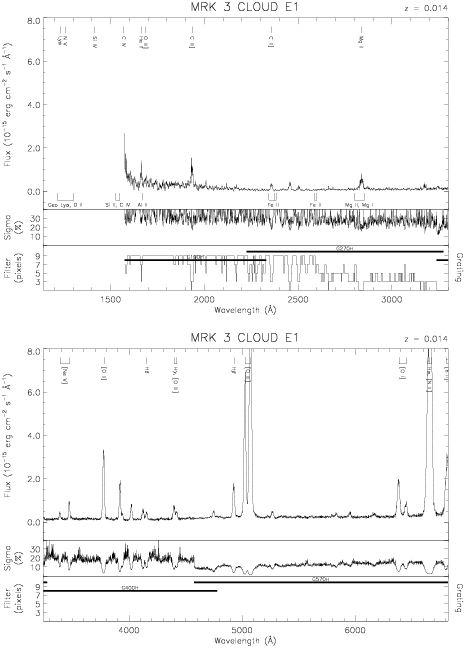Download dataset
TELESCOP= 'HST     '
INSTRUME= 'FOS     '
EQUINOX =               2000.0
DATE-OBS= '1993-09-23T07:18:21'
RA_PNT  =        93.9011666667
DEC_PNT =        71.0376833333
PA_PNT  =        33.9050279328
OBJECT  = 'MRK 3 CLOUD E1'
ROOTNM01= 'Y1GG0106T'
GRNDMD01= 'SPECTROSCOPY'
DETECT01= 'BLUE    '
APERID01= 'B-2     '
FGWAID01= 'H19     '
ROOTNM02= 'Y1GG0107T'
GRNDMD02= 'SPECTROSCOPY'
DETECT02= 'BLUE    '
APERID02= 'B-2     '
FGWAID02= 'H19     '
ROOTNM03= 'Y1HV0204T'
GRNDMD03= 'SPECTROSCOPY'
DETECT03= 'AMBER   '
APERID03= 'B-2     '
FGWAID03= 'H57     '
ROOTNM04= 'Y1HV0205T'
GRNDMD04= 'SPECTROSCOPY'
DETECT04= 'AMBER   '
APERID04= 'B-2     '
FGWAID04= 'H57     '
ROOTNM05= 'Y1HV0206T'
GRNDMD05= 'SPECTROSCOPY'
DETECT05= 'AMBER   '
APERID05= 'B-2     '
FGWAID05= 'H40     '
ROOTNM06= 'Y1HV0207T'
GRNDMD06= 'SPECTROSCOPY'
DETECT06= 'AMBER   '
APERID06= 'B-2     '
FGWAID06= 'H40     '
ROOTNM07= 'Y1HV0208T'
GRNDMD07= 'SPECTROSCOPY'
DETECT07= 'AMBER   '
APERID07= 'B-2     '
FGWAID07= 'H27     '